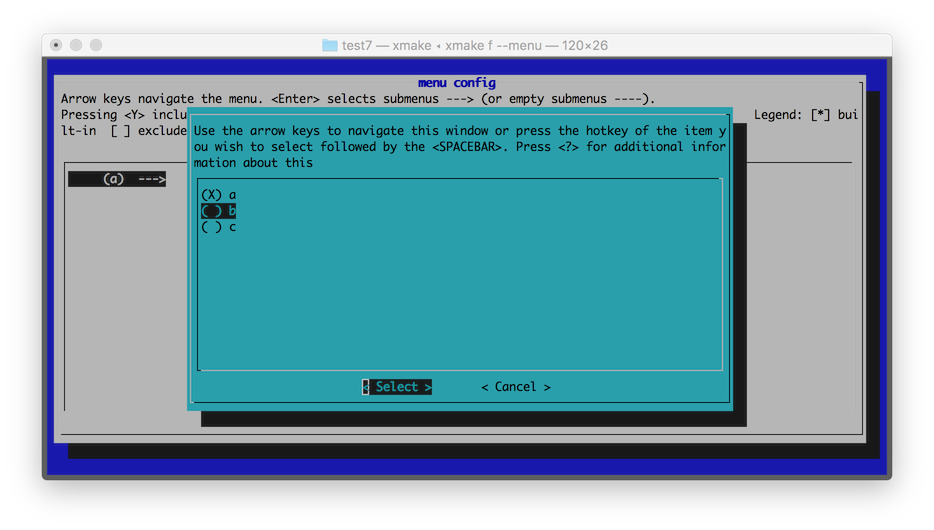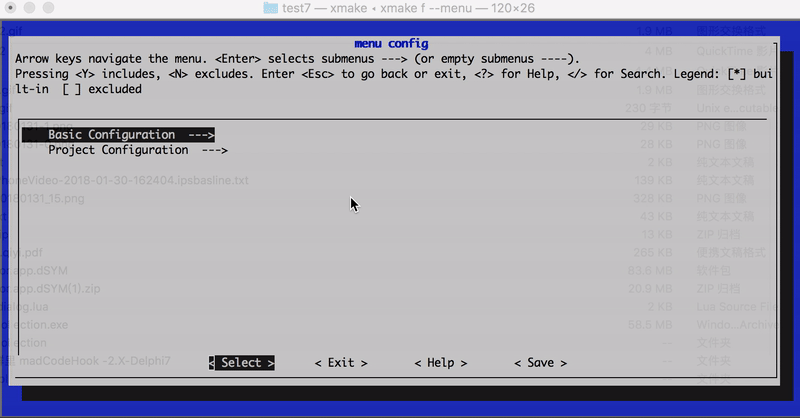#### This is a mirror page, please see the original page:

https://xmake.io/#/zh-cn/manual/configuration_option

``````-- 进入option的匿名全局域，里面的设置会同时影响test和test2选项
option()

option("test")
-- ...
-- 尽量保持缩进，因为这个之后的所有设置，都是针对test选项的

option("test2")
-- ...
``````

`option`域是可以重复进入来实现分离设置的，如果要显示离开当前选项的作用域设置，可以手动调用option_end接口。

option 定义选项 >= 2.0.1
option_end 结束定义选项 >= 2.1.1
before_check 选项检测之前执行此脚本 >= 2.1.5
on_check 自定义选项检测脚本 >= 2.1.5
after_check 选项检测之后执行此脚本 >= 2.1.5
set_values 设置选项值列表 >= 2.1.9
set_default 设置默认值 >= 2.0.1
set_category 设置选项分类，仅用于菜单显示 >= 1.0.1
set_description 设置菜单显示描述 >= 1.0.1
set_warnings 设置警告级别 >= 1.0.1
set_optimize 设置优化级别 >= 1.0.1
set_languages 设置代码语言标准 >= 1.0.1

### option

#### 定义选项

``````option("test")
set_default(false)
``````

``````target("demo")
``````

``````# 手动启用这个选项
\$ xmake f --test=y
\$ xmake
``````

### option_end

#### 添加选项依赖

``````option("small")
set_default(true)
on_check(function (option)
-- ...
end)

option("test")
set_default(true)
on_check(function (option)
if option:dep("small"):enabled() then
option:enable(false)
end
end)
``````

### option:before_check

#### 选项检测之前执行此脚本

``````option("zlib")
before_check(function (option)
import("lib.detect.find_package")
end)
``````

### option:on_check

#### 自定义选项检测脚本

``````option("test")
set_default(true)
on_check(function (option)
if option:dep("small"):enabled() then
option:enable(false)
end
end)
``````

### option:after_check

#### 选项检测之后执行此脚本

``````option("test")
after_check(function (option)
option:enable(false)
end)
``````

### option:set_values

#### 设置选项值列表

``````option("test")
set_default("b")
set_values("a", "b", "c")
``````### option:set_default

#### 设置选项默认值

``````option("test")
-- 默认禁用这个选项
set_default(false)
``````

``````option("test")
set_default("value")
``````

boolean 一般用作参数开关，值范围：`true/false` `xmake f --optionname=[y/n/yes/no/true/false]`
string 可以是任意字符串，一般用于模式判断 `xmake f --optionname=value`

``````-- 定义一个路径配置选项，默认使用临时目录
option("rootdir")
set_default("\$(tmpdir)")

target("test")
-- 添加指定选项目录中的源文件
``````

``````\$ xmake f --rootdir=~/projectdir/src
\$ xmake
``````

default值 检测行为

false 开关选项，不自动检测，默认禁用，可手动配置修改
true 开关选项，不自动检测，默认启用，可手动配置修改
string类型 无开关状态，不自动检测，可手动配置修改，一般用于配置变量传递

#### 设置是否启用菜单显示

``````option("test")
``````

``````Options:
...

--test=TEST
``````

### option:set_category

#### 设置选项分类，仅用于菜单显示

``````option("test1")
set_category("test")

option("test2")
set_category("test")

option("demo1")
set_category("demo")

option("demo2")
set_category("demo")
``````

``````Options:
...

--test1=TEST1
--test2=TEST2

--demo1=DEMO1
--demo2=DEMO2
``````

``````-- 'boolean' option
option("test1")
set_default(true)

-- 'choice' option with values: "a", "b", "c"
option("test2")
set_default("a")
set_values("a", "b", "c")

-- 'string' option
option("test3")
set_default("xx")

-- 'number' option
option("test4")
set_default(6)
``````

• test1
• test2
• test3
• test3
• test4### option:set_description

#### 设置菜单显示描述

``````option("test")
set_default(false)
set_description("Enable or disable test")
``````

``````Options:
...

--test=TEST                       Enable or disable test (default: false)
``````

``````option("mode")
set_default("debug")
set_description("Set build mode",
"    - debug",
"    - release",
"    - profile")
``````

``````Options:
...

--mode=MODE                       Set build mode (default: debug)
- debug
- release
- profile
``````

``````\$ xmake f --mode=release
``````

#### 添加链接库检测

``````option("pthread")
set_default(false)

target("test")
``````

#### 添加c头文件检测

``````option("pthread")
set_default(false)

target("test")
``````

#### 添加c类型检测

``````option("wchar")
set_default(false)

target("test")
``````

#### 添加c++代码片段检测

``````option("constexpr")
add_cxxsnippet("constexpr", "constexpr int f(int x) { int sum=0; for (int i=0; i<=x; ++i) sum += i; return sum; } constexpr int x = f(5);  static_assert(x == 15);")
``````